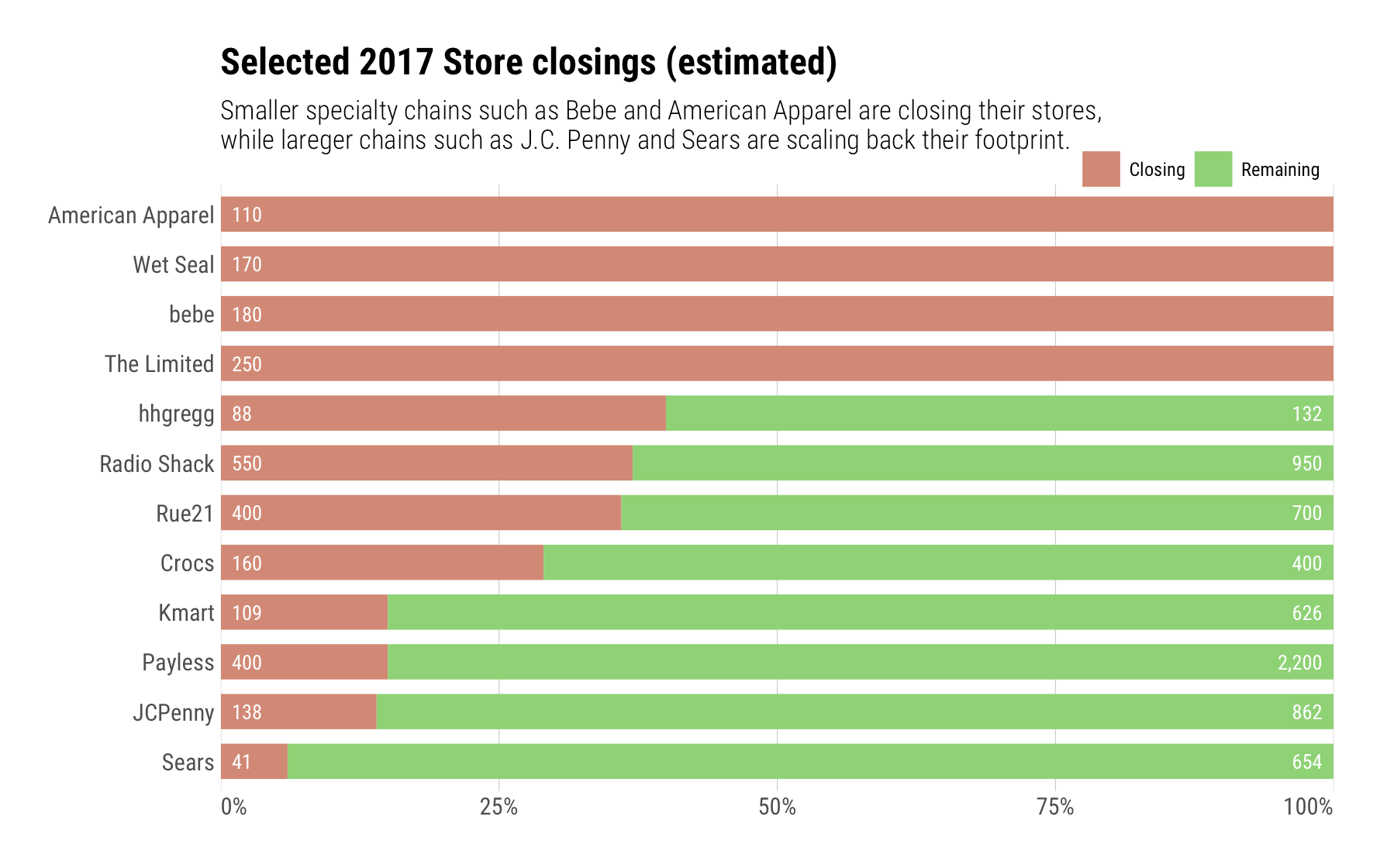# Shuttering Pies With Retiring Stores

I caught this “gem” in the Wall Street Journal tonight:It’s pretty hard to compare store-to-store, even though it is fairly clear which ones are going-going-gone. If we want to see the relative percentage of each store closing and also want to see how they stack up against each other, then let’s make a column of 100% bars and label total stores in each:

``````library(hrbrthemes)
library(tidyverse)

"Payless",400,2600
"Rue21",400,1100
"The Limited",250,250
"bebe",180,180
"Wet Seal",170,170
"Crocs",160,560
"JCPenny",138,1000
"American Apparel",110,110
"Kmart",109,735
"hhgregg",88,220
as_tibble() %>%
mutate(remaining = total - closing,
gone = round((closing/total) * 100)/100,
stay = 1-gone,
rem_lab = ifelse(remaining == 0, "", scales::comma(remaining))) %>%
arrange(desc(stay)) %>%
mutate(store=factor(store, levels=store)) -> closing_df

update_geom_font_defaults(font_rc)

ggplot(closing_df) +
geom_segment(aes(0, store, xend=gone, yend=store, color="Closing"), size=8) +
geom_segment(aes(gone, store, xend=gone+stay, yend=store, color="Remaining"), size=8) +
geom_text(aes(x=0, y=store, label=closing), color="white", hjust=0, nudge_x=0.01) +
geom_text(aes(x=1, y=store, label=rem_lab), color="white", hjust=1, nudge_x=-0.01) +
scale_x_percent() +
scale_color_ipsum(name=NULL) +
labs(x=NULL, y=NULL,
title="Selected 2017 Store closings (estimated)",
subtitle="Smaller specialty chains such as Bebe and American Apparel are closing their stores,\nwhile lareger chains such as J.C. Penny and Sears are scaling back their footprint.") +
theme_ipsum_rc(grid="X") +
theme(axis.text.x=element_text(hjust=c(0, 0.5, 0.5, 0.5, 1))) +
theme(legend.position=c(0.875, 1.025)) +
theme(legend.direction="horizontal")``````One might try circle packing or a treemap to show both relative store count and percentage, but I think the bigger story is the percent reduction for each retail chain. It’d be cool to see what others come up with.Amazon Author Page

## 7 Comments →Shuttering Pies With Retiring Stores

1.The Come On Man

library(ggplot2)
library(scales)

text=’store,closing,total
“Payless”,400,2600
“Rue21”,400,1100
“The Limited”,250,250
“bebe”,180,180
“Wet Seal”,170,170
“Crocs”,160,560
“JCPenny”,138,1000
“American Apparel”,110,110
“Kmart”,109,735
“hhgregg”,88,220
“Sears”,41,695′,
sep=”,”,
stringsAsFactors=FALSE
)

dtDataset = dtDataset[order(dtDataset\$total),]

dtDataset\$closingpct = dtDataset\$closing / dtDataset\$total

dtDataset\$cumtotal = cumsum(dtDataset\$total)
dtDataset\$prevcumtotal = dtDataset\$cumtotal – dtDataset\$total

ggplot() +
geomrect(
data = dtDataset,
aes(
xmin = prevcumtotal,
xmax = cumtotal,
ymin = 0,
ymax = closingpct,
fill = ‘Closing’
),
color = ‘black’
) +
geom
rect(
data = dtDataset,
aes(
xmin = prevcumtotal,
xmax = cumtotal,
ymin = closingpct,
ymax = 1,
fill = ‘Left’
),
color = ‘black’
) +
geomtext(
data = dtDataset,
aes(
x = prevcumtotal,
y = 1,
label = store
),
angle = 90,
hjust = 1,
vjust = 1,
nudge
y = -0.02,
nudgex = 0.02,
color = ‘black’
) +
scale
y_continuous(labels = percent, name = ‘% of Stores by Brand’) +
xlab(‘Total Stores Across Brands’)

2. Pingback: Shuttering Pies With Retiring Stores – Cyber Security

3.Antony Unwin

Good example and a nice graphic. You could make the bars proportional to the numbers of stores for each brand using ggmosaic:

library(tidyverse)
library(ggmosaic)
library(hrbrthemes)

dtD % mutate(open=total-closing, closingpct=closing/total*100)
dtx <- gather(dtD, status, number, closing, open)
dtx % mutate(Store = factor(reorder(store, closingpct)))

ggplot(data = dtx) + geommosaic(aes(x=product(factor(status), Store), weight=number, fill = factor(status)), offset=0.005, divider=mosaic(“h”)) + coordflip() + scalefillipsum(name = NULL) + scaleypercent() +
theme(legend.position=”bottom”, legend.title=elementblank(), plot.title = elementtext(hjust = 0.5)) +
labs(x=NULL, y=NULL,title=”Selected 2017 Store closings (estimated)”, subtitle=”Smaller specialty chains such as Bebe and American Apparel are closing their stores,\nwhile lareger chains such as J.C. Penny and Sears are scaling back their footprint.”)

(Unfortunately ggmosaic currently includes an offset for the 100% cases, does someone know how to get round this?)

4.Nguyen Nguyen

Instead of using geom_segment() we can use geom_col() for creating the above plot as follows:

http://rpubs.com/chidungkt/520807

1.hrbrmstr

Thx for reading & contributing! As the person who wrote `geom_col()`, I’m keenly aware of said geom :-) I use geom_segment() in its stead for lots of reasons across this and many other projects.

This site uses Akismet to reduce spam. Learn how your comment data is processed.